Games
Problems
Go Pro!

The question of the day is:Can you solve it? If you said "yes," Then you are probably wrong. This is really not a "solvable" problem. It was created by someone who wanted to generate arguments and disagreements, because arguments translate into attention, likes, and shares.

You see, it's pretty much impossible to draw conclusions about a pattern when you only have one example from which to draw your conclusions. Depending on what pattern you notice, there are a multitude of "correct" answers.

I think the most common conclusion is: 5 is the number of squares in the image (four little ones and one big one). If this was your interpretation, then the answer is 14 (9 little squares, four medium squares, one large square).

However, there are other ways of interpreting the image. 5 is the number of line intersections involving interior line segments. In which case, the number for the second image is 12.

Another way of looking at it: 5 is the number of squares which border on the edge of the diagram. In which case, the answer to the second diagram is 13 instead of 14, because one of the squares (the central little square) doesn't border on the edge of the diagram.

But we don't have to stop there - as Brian Twitchell (District Math Coach, RSU #74) points out, we can create even more patterns:

• If we take one less than the number of horizontal unit segments, we end up with an answer of 11.
• If we take seven less than the sum of the number of horizontal and vertical unit segments, we get an answer of 17.
• Forget unit segments; consider line segments that span the length/height of the diagram. Now subtract one. This also matches the original diagram, and gives an answer of 7.

So don't be too quick to assume you have "the" answer to a question!

If you enjoyed this post, you might also be interested in Professor Puzzler's analysis of this sneaky problem: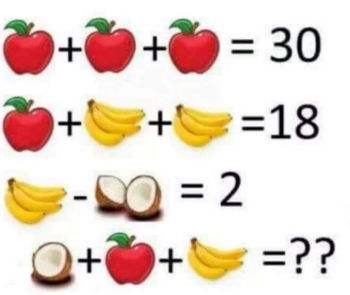The image below has been showing up in various incarnations on the internet. Sometimes with an image of Albert Einstein, sometimes with an image of Sheldon from "Big Bang Theory."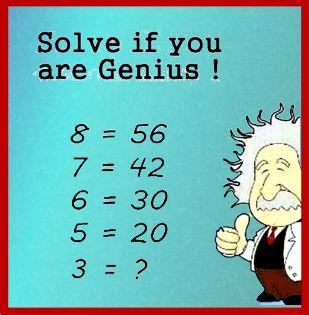I especially find this meme ridiculous when it's accompanied by a picture of Sheldon posing this as a problem for Penny, because anyone who knows Sheldon, and knows anything about math, know perfectly well that Sheldon would never, ever, ever pose a problem like this. Instead, he would, like good ol' Albert in the image below, give it an emphatic thumbs down.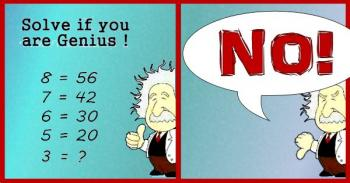What would Sheldon say the answer is?

He would say, "Three. The answer is three. Bazinga!"

Einstein probably wouldn't say "Bazinga," but he would agree. The answer is three.

"But," someone might protest to Sheldon, "That doesn't fit the pattern!"

"Oh," Sheldon would say, "You want a pattern? Okay. The answer is the square root of seventeen."

"No," you would say, "That's wrong."

"Exactly," Sheldon would say. "You wanted me to create a pattern. The first four equations are wrong, so I made another wrong equation to complete the pattern. Bazinga again!"

Einstein would probably answer differently. He would probably say, "Just because the first four equations are wrong doesn't mean I have to be wrong, too. The answer is three."

You see, in mathematics, the equals sign has a very specific meaning; it means that the two sides of the equation are equal. So I don't care if the person who made this meme got four equations wrong, the answer is still three.

What the problem writer probably meant to do was to use function notation:

f(8) = 56
f(7) = 42
f(6) = 30
f(5) = 20
f(3) = ?

Now we at least have valid mathematical notation. But is the problem solvable? Not really, no. Because there isn't just one function which satisfies the values above, and not all of them produce the same value for f(3).

For example, the function that the image creator probably wanted you to use was: f(x) = x- x, which works for all the values given, and gives f(3) = 32 - 3 = 6. This is the answer most people are accepting as correct. However, consider the following as an alternative function:

f(x) = (x3 - 4x+ 3x)/(x - 3)

With this function, we get the correct values but we can't plug three into the function because it results in a divsion by zero!

So, even if we fix the notation, the real geniuses will tell you that it can't be solved.

Bazinga.

• Phil wanted to know if I could find a function that matched all the data points in the pattern. but produced a different value for f(3). (Apparently he thought it was cheating to create a function that has no value for f(3)!). Anyway, the answer is, yes, I can do it, and here's the explanation of how: Creating Einstein's Meme Function.
• Toward the end here I'm being a bit tongue in cheek, pointing out that there isn't just one solution. For most of us, though, if we can find a pattern that matches four data points, we feel like we've come up with a satisfactory "solution."  It's a whole different matter if you're given only one data point and asked to find a pattern, which is what happens in the puzzle linked below. Be sure to read about the "squares" problem for more about that!

Click the image below for another question and answer!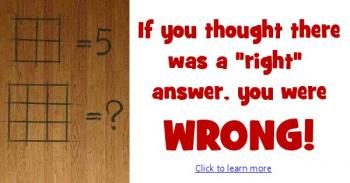What is the solution to the following puzzle that's been going around facebook?The answer is, on the surface, not terribly difficult to find:

3 apples = 30, so 1 apple = 10.

10 + 2 bananas = 18, so 2 bananas = 8 and 1 banana = 4

4 - 1 coconut = 2, so 1 coconut = 2

Therefore, 1 coconut + 1 apple + 1 banana = 2 + 10 + 4 = 16

## HOWEVER...

Although this is the answer everyone seems to be giving, it's actually not correct. Notice that each bunch of bananas has four bananas (look closely!) except the one in the final line, which has 3 bananas. Also notice that the third line has one coconut, while the last line has only 1/2 of a coconut.

This changes things!

3 apples = 30, so 1 apple = 10.

10 + 8 bananas = 18, so 8 bananas = 8 and 1 banana = 1

4 - 1 coconut = 2, so 1 coconut = 2

Therefore, 1/2 coconut + 1 apple + 3 bananas = 1 + 10 + 3 = 14

This is a really nice problem, since it gives you a good reminder to pay attention to details!

Click the image below to read Professor Puzzler's analysis of another puzzle:William from Phoenix asks, "Apples coconuts bananas: The editing to remove the fourth banana was incomplete. it left the end point in the picture on line four. Was that intentional to cause controversy with your answers?"

Honestly, William, I can't see the incomplete erasure you're talking about. I don't doubt you, but I'm not seeing it. But, since you asked, I'll take this opportunity to point out: I didn't create this problem; I just wrote up the solution for people who wanted to know. If I had to make a guess, I would say that the person who created it did not intentionally leave part of a banana; they probably were just inexperienced with photoshop, or were in a hurry when they created it!

Colton from Florida had a question about the Algebra of this problem, which we answered here: Can X and Y have the same value?

Pany from UK points out that there is another way to interpret the problem, in which we regard each image as a symbolic variable, rather than as collections of real world objects. While I don't agree with him that this is the best way of looking at it, it is absolutely a valid way of viewing the problem, and it results in a very different problem, which is unsolvable, because we have more variables than equations. And speaking of which...

Anne from Maine shared with us a similar looking problem with a very different solution. Click the image below to read that post: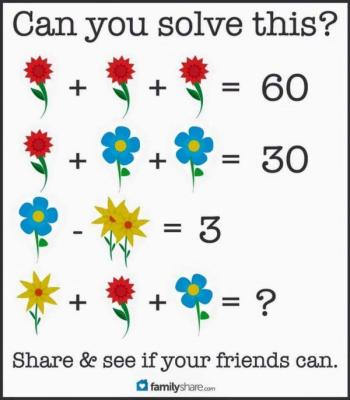# Featured Resources on This Site

Create Christmas ornaments and more
Print fun mazes in many different shapes

# Blogs on This SiteReviews and book lists - books we love!The site administrator fields questions from visitors.Like us on Facebook to get updates about new resources